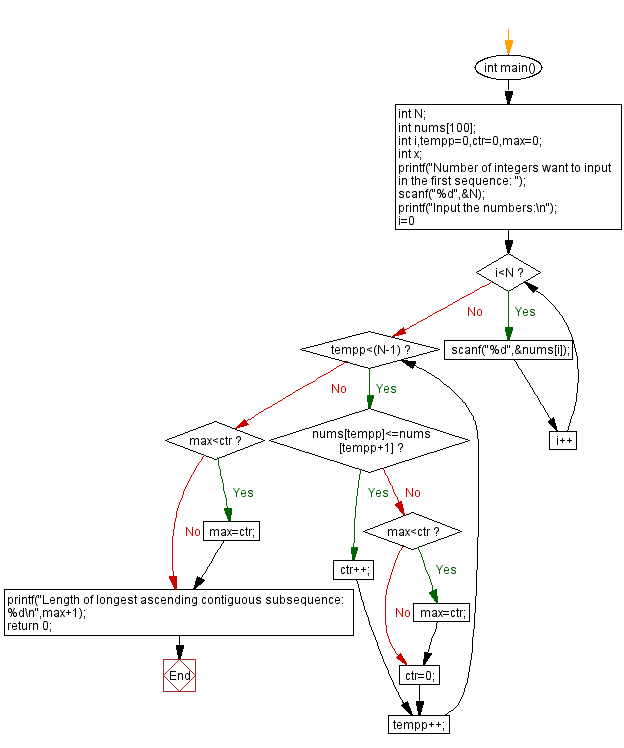﻿ C - Length of longest ascending contiguous subsequence

# C Exercises: Length of longest ascending contiguous subsequence

## C Basic-II: Exercise-6 with Solution

Write a C program that accept a sequence of positive integers from the user and find the length of the longest continuous subsequence from the said sequence.

Sample Date:

Length of the sequence: 5 Sequence: 5 2 3 4 1 Length of longest ascending contiguous subsequence: 5 [2 3 4] Length of the sequence: 6 Sequence: 10 20 30 40 50 60 Length of longest ascending contiguous subsequence: 6 [10 20 30 40 50 60] Length of the sequence: 3 Sequence: 5 1 3 Length of longest ascending contiguous subsequence: 2 [1 3]

C Code:

``````#include <stdio.h>
int main()
{
int N;
int nums;
int i,tempp=0,ctr=0,max=0;
int x;
printf("Number of integers want to input in the first sequence: ");
scanf("%d",&N);
printf("Input the numbers:\n");
for(i=0;i<N;i++){
scanf("%d",&nums[i]);
}
while(tempp<(N-1))
{
if(nums[tempp]<=nums[tempp+1])
{
ctr++;
}
else
{
if(max<ctr) max=ctr;
ctr=0;
}
tempp++;
}
if(max<ctr)
max=ctr;
printf("Length of longest ascending contiguous subsequence: %d\n",max+1);
return 0;
}
``````

Sample Output:

```Number of integers want to input in the first sequence: 5
Input the numbers:
5 2 3 4 1
Length of longest ascending contiguous subsequence: 3
```

Flowchart:C Programming Code Editor:

Previous C Programming Exercise: Sum values before, after the maximum value in a sequence.
Next C Programming Exercise: The smallest absolute difference between X and 2 integers.

What is the difficulty level of this exercise?

Test your Programming skills with w3resource's quiz.

﻿

```MY_TYPE a = { .flag = true, .value = 123, .stuff = 0.456 };